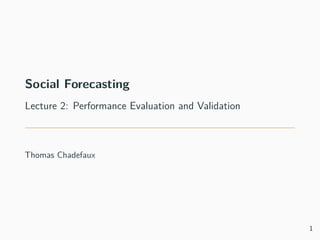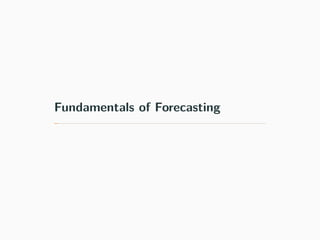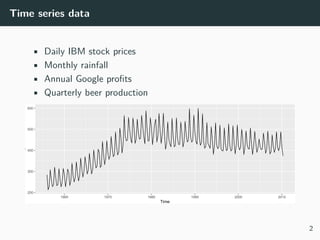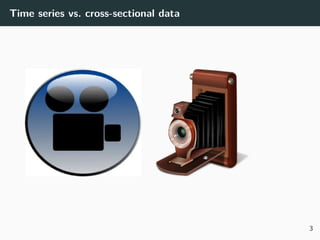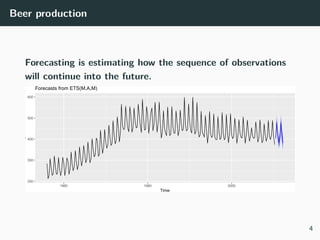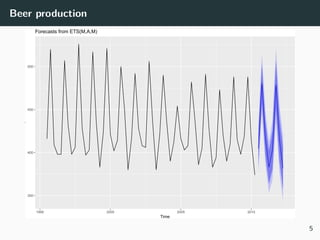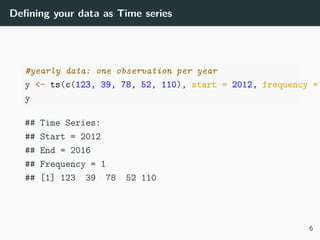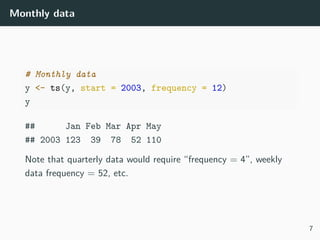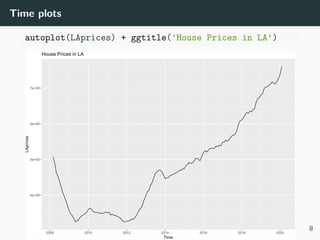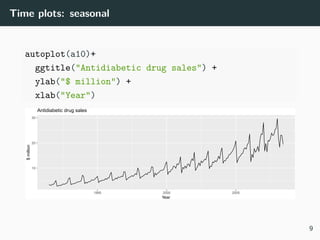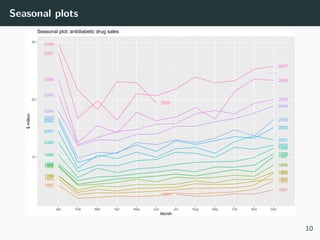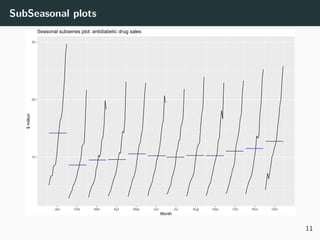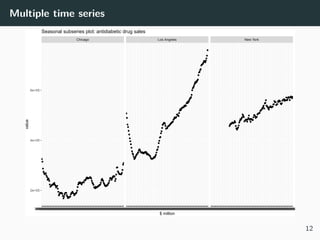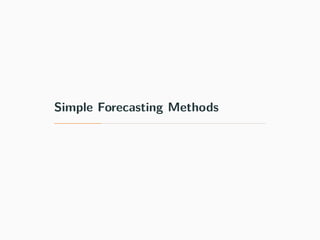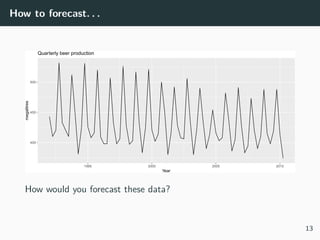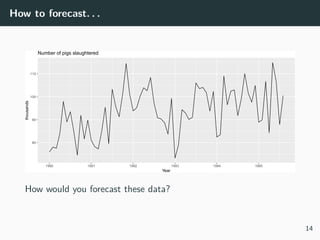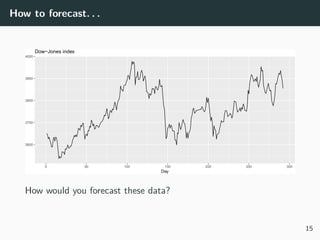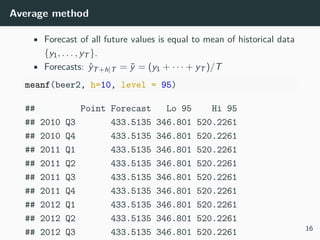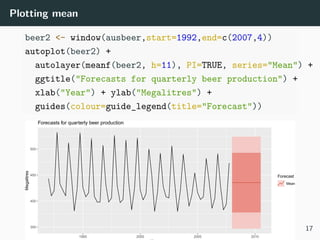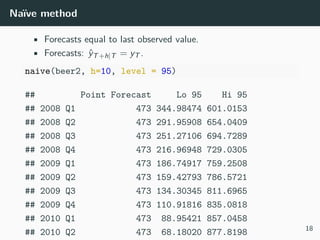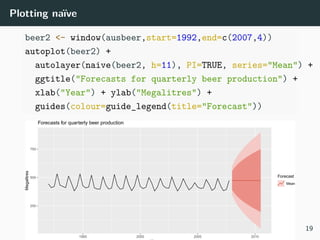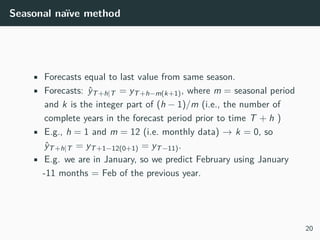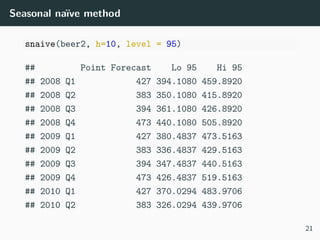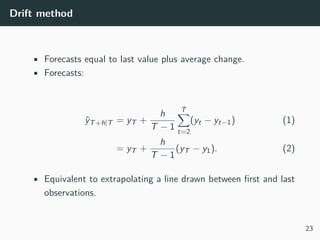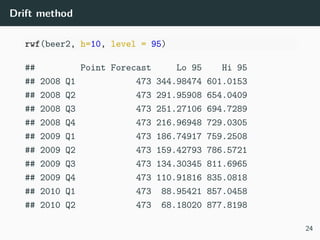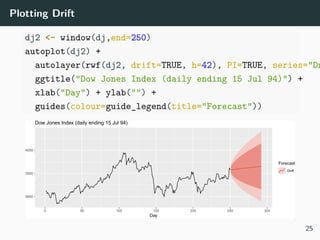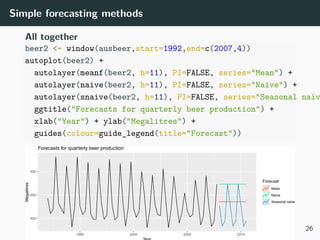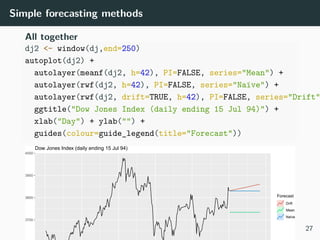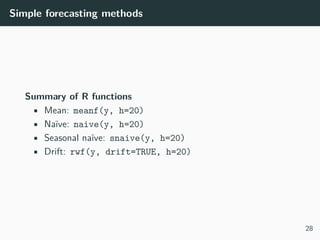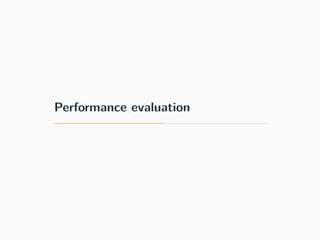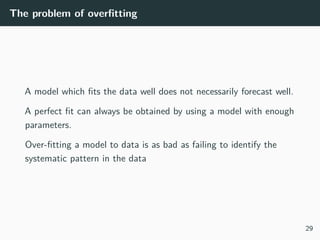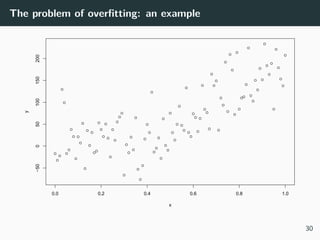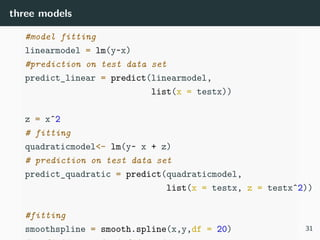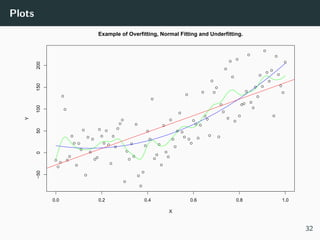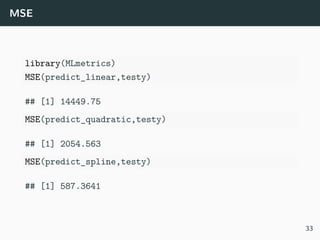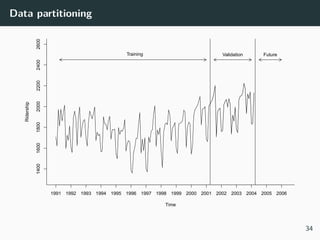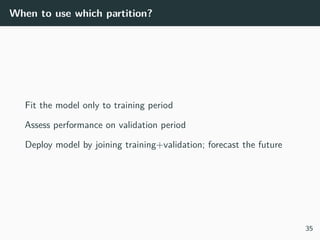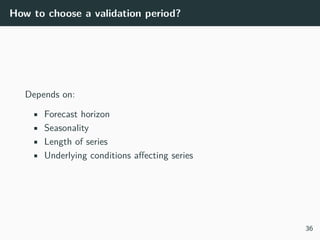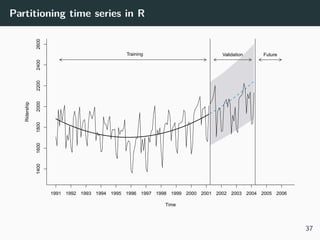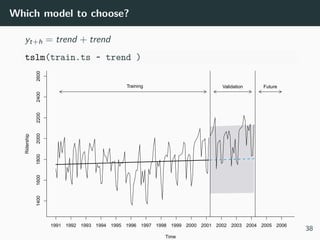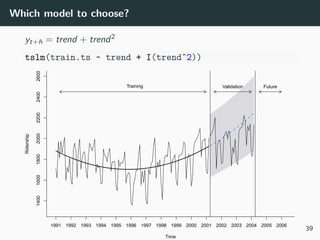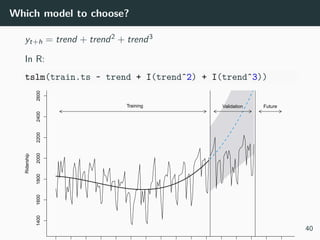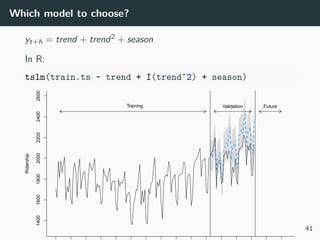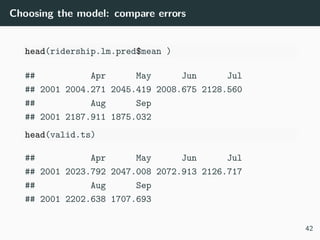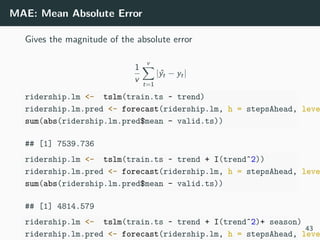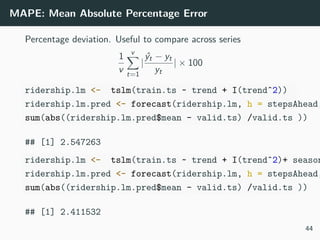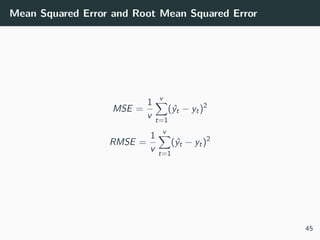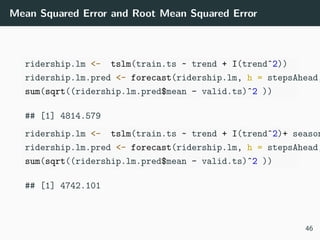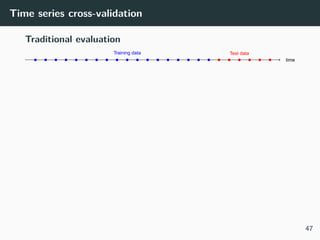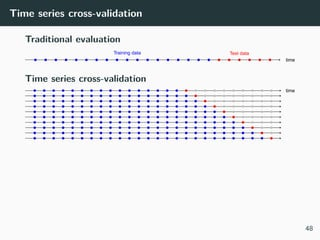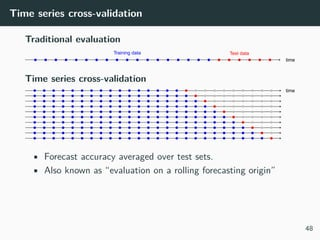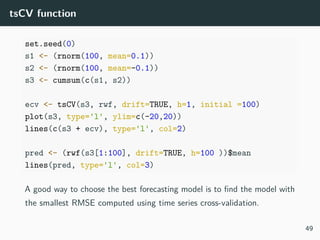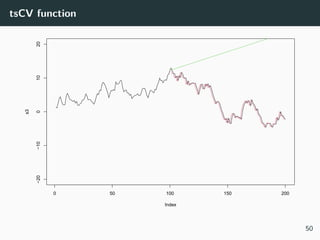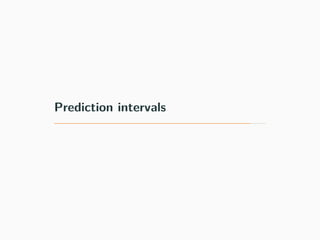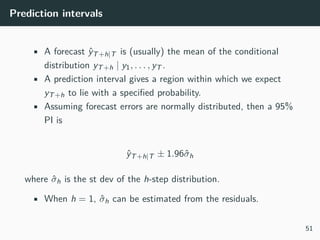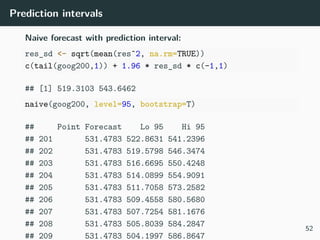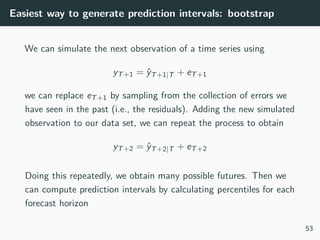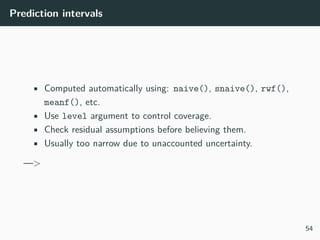1 of 59

### lecture2.pdf

1. Social Forecasting Lecture 2: Performance Evaluation and Validation Thomas Chadefaux 1
2. Fundamentals of Forecasting
3. Time series data • Daily IBM stock prices • Monthly rainfall • Annual Google profits • Quarterly beer production 200 300 400 500 600 1960 1970 1980 1990 2000 2010 Time . 2
4. Time series vs. cross-sectional data 3
5. Beer production Forecasting is estimating how the sequence of observations will continue into the future. 200 300 400 500 600 1960 1980 2000 Time . Forecasts from ETS(M,A,M) 4
6. Beer production 350 400 450 500 1995 2000 2005 2010 Time . Forecasts from ETS(M,A,M) 5
7. Defining your data as Time series #yearly data: one observation per year y <- ts(c(123, 39, 78, 52, 110), start = 2012, frequency = y ## Time Series: ## Start = 2012 ## End = 2016 ## Frequency = 1 ##  123 39 78 52 110 6
8. Monthly data # Monthly data y <- ts(y, start = 2003, frequency = 12) y ## Jan Feb Mar Apr May ## 2003 123 39 78 52 110 Note that quarterly data would require “frequency = 4”, weekly data frequency = 52, etc. 7
9. Time plots autoplot(LAprices) + ggtitle('House Prices in LA') 4e+05 5e+05 6e+05 7e+05 2008 2010 2012 2014 2016 2018 2020 Time LAprices House Prices in LA 8
10. Time plots: seasonal autoplot(a10)+ ggtitle("Antidiabetic drug sales") + ylab("\$ million") + xlab("Year") 10 20 30 1995 2000 2005 Year \$ million Antidiabetic drug sales 9
11. Seasonal plots 1991 1991 1992 1992 1993 1993 1994 1994 1995 1995 1996 1996 1997 1997 1998 1998 1999 1999 2000 2000 2001 2001 2002 2002 2003 2003 2004 2004 2005 2005 2006 2006 2007 2007 2008 2008 10 20 30 Jan Feb Mar Apr May Jun Jul Aug Sep Oct Nov Dec Month \$ million Seasonal plot: antidiabetic drug sales 10
12. SubSeasonal plots 10 20 30 Jan Feb Mar Apr May Jun Jul Aug Sep Oct Nov Dec Month \$ million Seasonal subseries plot: antidiabetic drug sales 11
13. Multiple time series Chicago Los Angeles New York X2008.03 X2008.04 X2008.05 X2008.06 X2008.07 X2008.08 X2008.09 X2008.10 X2008.11 X2008.12 X2009.01 X2009.02 X2009.03 X2009.04 X2009.05 X2009.06 X2009.07 X2009.08 X2009.09 X2009.10 X2009.11 X2009.12 X2010.01 X2010.02 X2010.03 X2010.04 X2010.05 X2010.06 X2010.07 X2010.08 X2010.09 X2010.10 X2010.11 X2010.12 X2011.01 X2011.02 X2011.03 X2011.04 X2011.05 X2011.06 X2011.07 X2011.08 X2011.09 X2011.10 X2011.11 X2011.12 X2012.01 X2012.02 X2012.03 X2012.04 X2012.05 X2012.06 X2012.07 X2012.08 X2012.09 X2012.10 X2012.11 X2012.12 X2013.01 X2013.02 X2013.03 X2013.04 X2013.05 X2013.06 X2013.07 X2013.08 X2013.09 X2013.10 X2013.11 X2013.12 X2014.01 X2014.02 X2014.03 X2014.04 X2014.05 X2014.06 X2014.07 X2014.08 X2014.09 X2014.10 X2014.11 X2014.12 X2015.01 X2015.02 X2015.03 X2015.04 X2015.05 X2015.06 X2015.07 X2015.08 X2015.09 X2015.10 X2015.11 X2015.12 X2016.01 X2016.02 X2016.03 X2016.04 X2016.05 X2016.06 X2016.07 X2016.08 X2016.09 X2016.10 X2016.11 X2016.12 X2017.01 X2017.02 X2017.03 X2017.04 X2017.05 X2017.06 X2017.07 X2017.08 X2017.09 X2017.10 X2017.11 X2017.12 X2018.01 X2018.02 X2018.03 X2018.04 X2018.05 X2018.06 X2018.07 X2018.08 X2018.09 X2018.10 X2018.11 X2018.12 X2019.01 X2019.02 X2019.03 X2019.04 X2019.05 X2019.06 X2019.07 X2019.08 X2019.09 X2019.10 X2019.11 X2019.12 X2020.01 X2020.02 X2020.03 X2008.03 X2008.04 X2008.05 X2008.06 X2008.07 X2008.08 X2008.09 X2008.10 X2008.11 X2008.12 X2009.01 X2009.02 X2009.03 X2009.04 X2009.05 X2009.06 X2009.07 X2009.08 X2009.09 X2009.10 X2009.11 X2009.12 X2010.01 X2010.02 X2010.03 X2010.04 X2010.05 X2010.06 X2010.07 X2010.08 X2010.09 X2010.10 X2010.11 X2010.12 X2011.01 X2011.02 X2011.03 X2011.04 X2011.05 X2011.06 X2011.07 X2011.08 X2011.09 X2011.10 X2011.11 X2011.12 X2012.01 X2012.02 X2012.03 X2012.04 X2012.05 X2012.06 X2012.07 X2012.08 X2012.09 X2012.10 X2012.11 X2012.12 X2013.01 X2013.02 X2013.03 X2013.04 X2013.05 X2013.06 X2013.07 X2013.08 X2013.09 X2013.10 X2013.11 X2013.12 X2014.01 X2014.02 X2014.03 X2014.04 X2014.05 X2014.06 X2014.07 X2014.08 X2014.09 X2014.10 X2014.11 X2014.12 X2015.01 X2015.02 X2015.03 X2015.04 X2015.05 X2015.06 X2015.07 X2015.08 X2015.09 X2015.10 X2015.11 X2015.12 X2016.01 X2016.02 X2016.03 X2016.04 X2016.05 X2016.06 X2016.07 X2016.08 X2016.09 X2016.10 X2016.11 X2016.12 X2017.01 X2017.02 X2017.03 X2017.04 X2017.05 X2017.06 X2017.07 X2017.08 X2017.09 X2017.10 X2017.11 X2017.12 X2018.01 X2018.02 X2018.03 X2018.04 X2018.05 X2018.06 X2018.07 X2018.08 X2018.09 X2018.10 X2018.11 X2018.12 X2019.01 X2019.02 X2019.03 X2019.04 X2019.05 X2019.06 X2019.07 X2019.08 X2019.09 X2019.10 X2019.11 X2019.12 X2020.01 X2020.02 X2020.03 X2008.03 X2008.04 X2008.05 X2008.06 X2008.07 X2008.08 X2008.09 X2008.10 X2008.11 X2008.12 X2009.01 X2009.02 X2009.03 X2009.04 X2009.05 X2009.06 X2009.07 X2009.08 X2009.09 X2009.10 X2009.11 X2009.12 X2010.01 X2010.02 X2010.03 X2010.04 X2010.05 X2010.06 X2010.07 X2010.08 X2010.09 X2010.10 X2010.11 X2010.12 X2011.01 X2011.02 X2011.03 X2011.04 X2011.05 X2011.06 X2011.07 X2011.08 X2011.09 X2011.10 X2011.11 X2011.12 X2012.01 X2012.02 X2012.03 X2012.04 X2012.05 X2012.06 X2012.07 X2012.08 X2012.09 X2012.10 X2012.11 X2012.12 X2013.01 X2013.02 X2013.03 X2013.04 X2013.05 X2013.06 X2013.07 X2013.08 X2013.09 X2013.10 X2013.11 X2013.12 X2014.01 X2014.02 X2014.03 X2014.04 X2014.05 X2014.06 X2014.07 X2014.08 X2014.09 X2014.10 X2014.11 X2014.12 X2015.01 X2015.02 X2015.03 X2015.04 X2015.05 X2015.06 X2015.07 X2015.08 X2015.09 X2015.10 X2015.11 X2015.12 X2016.01 X2016.02 X2016.03 X2016.04 X2016.05 X2016.06 X2016.07 X2016.08 X2016.09 X2016.10 X2016.11 X2016.12 X2017.01 X2017.02 X2017.03 X2017.04 X2017.05 X2017.06 X2017.07 X2017.08 X2017.09 X2017.10 X2017.11 X2017.12 X2018.01 X2018.02 X2018.03 X2018.04 X2018.05 X2018.06 X2018.07 X2018.08 X2018.09 X2018.10 X2018.11 X2018.12 X2019.01 X2019.02 X2019.03 X2019.04 X2019.05 X2019.06 X2019.07 X2019.08 X2019.09 X2019.10 X2019.11 X2019.12 X2020.01 X2020.02 X2020.03 2e+05 4e+05 6e+05 \$ million value Seasonal subseries plot: antidiabetic drug sales 12
14. Simple Forecasting Methods
15. How to forecast. . . 400 450 500 1995 2000 2005 2010 Year megalitres Quarterly beer production How would you forecast these data? 13
16. How to forecast. . . 80 90 100 110 1990 1991 1992 1993 1994 1995 Year thousands Number of pigs slaughtered How would you forecast these data? 14
17. How to forecast. . . 3600 3700 3800 3900 4000 0 50 100 150 200 250 300 Day Dow−Jones index How would you forecast these data? 15
18. Average method • Forecast of all future values is equal to mean of historical data {y1, . . . , yT }. • Forecasts: ŷT+h|T = ȳ = (y1 + · · · + yT )/T meanf(beer2, h=10, level = 95) ## Point Forecast Lo 95 Hi 95 ## 2010 Q3 433.5135 346.801 520.2261 ## 2010 Q4 433.5135 346.801 520.2261 ## 2011 Q1 433.5135 346.801 520.2261 ## 2011 Q2 433.5135 346.801 520.2261 ## 2011 Q3 433.5135 346.801 520.2261 ## 2011 Q4 433.5135 346.801 520.2261 ## 2012 Q1 433.5135 346.801 520.2261 ## 2012 Q2 433.5135 346.801 520.2261 ## 2012 Q3 433.5135 346.801 520.2261 16
19. Plotting mean beer2 <- window(ausbeer,start=1992,end=c(2007,4)) autoplot(beer2) + autolayer(meanf(beer2, h=11), PI=TRUE, series="Mean") + ggtitle("Forecasts for quarterly beer production") + xlab("Year") + ylab("Megalitres") + guides(colour=guide_legend(title="Forecast")) 350 400 450 500 1995 2000 2005 2010 Megalitres Forecast Mean Forecasts for quarterly beer production 17
20. Naïve method • Forecasts equal to last observed value. • Forecasts: ŷT+h|T = yT . naive(beer2, h=10, level = 95) ## Point Forecast Lo 95 Hi 95 ## 2008 Q1 473 344.98474 601.0153 ## 2008 Q2 473 291.95908 654.0409 ## 2008 Q3 473 251.27106 694.7289 ## 2008 Q4 473 216.96948 729.0305 ## 2009 Q1 473 186.74917 759.2508 ## 2009 Q2 473 159.42793 786.5721 ## 2009 Q3 473 134.30345 811.6965 ## 2009 Q4 473 110.91816 835.0818 ## 2010 Q1 473 88.95421 857.0458 ## 2010 Q2 473 68.18020 877.8198 18
21. Plotting naïve beer2 <- window(ausbeer,start=1992,end=c(2007,4)) autoplot(beer2) + autolayer(naive(beer2, h=11), PI=TRUE, series="Mean") + ggtitle("Forecasts for quarterly beer production") + xlab("Year") + ylab("Megalitres") + guides(colour=guide_legend(title="Forecast")) 250 500 750 1995 2000 2005 2010 Megalitres Forecast Mean Forecasts for quarterly beer production 19
22. Seasonal naïve method • Forecasts equal to last value from same season. • Forecasts: ŷT+h|T = yT+h−m(k+1), where m = seasonal period and k is the integer part of (h − 1)/m (i.e., the number of complete years in the forecast period prior to time T + h ) • E.g., h = 1 and m = 12 (i.e. monthly data) → k = 0, so ŷT+h|T = yT+1−12(0+1) = yT−11). • E.g. we are in January, so we predict February using January -11 months = Feb of the previous year. 20
23. Seasonal naïve method snaive(beer2, h=10, level = 95) ## Point Forecast Lo 95 Hi 95 ## 2008 Q1 427 394.1080 459.8920 ## 2008 Q2 383 350.1080 415.8920 ## 2008 Q3 394 361.1080 426.8920 ## 2008 Q4 473 440.1080 505.8920 ## 2009 Q1 427 380.4837 473.5163 ## 2009 Q2 383 336.4837 429.5163 ## 2009 Q3 394 347.4837 440.5163 ## 2009 Q4 473 426.4837 519.5163 ## 2010 Q1 427 370.0294 483.9706 ## 2010 Q2 383 326.0294 439.9706 21
24. Plotting seasonal naive beer2 <- window(ausbeer,start=1992,end=c(2007,4)) autoplot(beer2) + autolayer(snaive(beer2, h=11), PI=TRUE, series="Seasonal ggtitle("Forecasts for quarterly beer production") + xlab("Year") + ylab("Megalitres") + guides(colour=guide_legend(title="Forecast")) 350 400 450 500 1995 2000 2005 2010 Megalitres Forecast Seasonal naïve Forecasts for quarterly beer production 22
25. Drift method • Forecasts equal to last value plus average change. • Forecasts: ŷT+h|T = yT + h T − 1 T X t=2 (yt − yt−1) (1) = yT + h T − 1 (yT − y1). (2) • Equivalent to extrapolating a line drawn between first and last observations. 23
26. Drift method rwf(beer2, h=10, level = 95) ## Point Forecast Lo 95 Hi 95 ## 2008 Q1 473 344.98474 601.0153 ## 2008 Q2 473 291.95908 654.0409 ## 2008 Q3 473 251.27106 694.7289 ## 2008 Q4 473 216.96948 729.0305 ## 2009 Q1 473 186.74917 759.2508 ## 2009 Q2 473 159.42793 786.5721 ## 2009 Q3 473 134.30345 811.6965 ## 2009 Q4 473 110.91816 835.0818 ## 2010 Q1 473 88.95421 857.0458 ## 2010 Q2 473 68.18020 877.8198 24
27. Plotting Drift dj2 <- window(dj,end=250) autoplot(dj2) + autolayer(rwf(dj2, drift=TRUE, h=42), PI=TRUE, series="Dr ggtitle("Dow Jones Index (daily ending 15 Jul 94)") + xlab("Day") + ylab("") + guides(colour=guide_legend(title="Forecast")) 3600 3800 4000 0 50 100 150 200 250 300 Day Forecast Drift Dow Jones Index (daily ending 15 Jul 94) 25
28. Simple forecasting methods All together beer2 <- window(ausbeer,start=1992,end=c(2007,4)) autoplot(beer2) + autolayer(meanf(beer2, h=11), PI=FALSE, series="Mean") + autolayer(naive(beer2, h=11), PI=FALSE, series="Naïve") + autolayer(snaive(beer2, h=11), PI=FALSE, series="Seasonal naïv ggtitle("Forecasts for quarterly beer production") + xlab("Year") + ylab("Megalitres") + guides(colour=guide_legend(title="Forecast")) 400 450 500 1995 2000 2005 2010 Megalitres Forecast Mean Naïve Seasonal naïve Forecasts for quarterly beer production 26
29. Simple forecasting methods All together dj2 <- window(dj,end=250) autoplot(dj2) + autolayer(meanf(dj2, h=42), PI=FALSE, series="Mean") + autolayer(rwf(dj2, h=42), PI=FALSE, series="Naïve") + autolayer(rwf(dj2, drift=TRUE, h=42), PI=FALSE, series="Drift" ggtitle("Dow Jones Index (daily ending 15 Jul 94)") + xlab("Day") + ylab("") + guides(colour=guide_legend(title="Forecast")) 3700 3800 3900 4000 Forecast Drift Mean Naïve Dow Jones Index (daily ending 15 Jul 94) 27
30. Simple forecasting methods Summary of R functions • Mean: meanf(y, h=20) • Naïve: naive(y, h=20) • Seasonal naïve: snaive(y, h=20) • Drift: rwf(y, drift=TRUE, h=20) 28
31. Performance evaluation
32. The problem of overfitting A model which fits the data well does not necessarily forecast well. A perfect fit can always be obtained by using a model with enough parameters. Over-fitting a model to data is as bad as failing to identify the systematic pattern in the data 29
33. The problem of overfitting: an example 0.0 0.2 0.4 0.6 0.8 1.0 −50 0 50 100 150 200 x y 30
34. three models #model fitting linearmodel = lm(y~x) #prediction on test data set predict_linear = predict(linearmodel, list(x = testx)) z = xˆ2 # fitting quadraticmodel<- lm(y~ x + z) # prediction on test data set predict_quadratic = predict(quadraticmodel, list(x = testx, z = testxˆ2)) #fitting smoothspline = smooth.spline(x,y,df = 20) 31
35. Plots 0.0 0.2 0.4 0.6 0.8 1.0 −50 0 50 100 150 200 Example of Overfitting, Normal Fitting and Underfitting. X Y 32
36. MSE library(MLmetrics) MSE(predict_linear,testy) ##  14449.75 MSE(predict_quadratic,testy) ##  2054.563 MSE(predict_spline,testy) ##  587.3641 33
37. Data partitioning Time Ridership 1400 1600 1800 2000 2200 2400 2600 1991 1992 1993 1994 1995 1996 1997 1998 1999 2000 2001 2002 2003 2004 2005 2006 Training Validation Future 34
38. When to use which partition? Fit the model only to training period Assess performance on validation period Deploy model by joining training+validation; forecast the future 35
39. How to choose a validation period? Depends on: • Forecast horizon • Seasonality • Length of series • Underlying conditions affecting series 36
40. Partitioning time series in R Time Ridership 1400 1600 1800 2000 2200 2400 2600 1991 1992 1993 1994 1995 1996 1997 1998 1999 2000 2001 2002 2003 2004 2005 2006 Training Validation Future 37
41. Which model to choose? yt+h = trend + trend tslm(train.ts ~ trend ) Time Ridership 1400 1600 1800 2000 2200 2400 2600 1991 1992 1993 1994 1995 1996 1997 1998 1999 2000 2001 2002 2003 2004 2005 2006 Training Validation Future 38
42. Which model to choose? yt+h = trend + trend2 tslm(train.ts ~ trend + I(trendˆ2)) Time Ridership 1400 1600 1800 2000 2200 2400 2600 1991 1992 1993 1994 1995 1996 1997 1998 1999 2000 2001 2002 2003 2004 2005 2006 Training Validation Future 39
43. Which model to choose? yt+h = trend + trend2 + trend3 In R: tslm(train.ts ~ trend + I(trendˆ2) + I(trendˆ3)) Ridership 1400 1600 1800 2000 2200 2400 2600 Training Validation Future 40
44. Which model to choose? yt+h = trend + trend2 + season In R: tslm(train.ts ~ trend + I(trendˆ2) + season) Ridership 1400 1600 1800 2000 2200 2400 2600 Training Validation Future 41
45. Choosing the model: compare errors head(ridership.lm.pred\$mean ) ## Apr May Jun Jul ## 2001 2004.271 2045.419 2008.675 2128.560 ## Aug Sep ## 2001 2187.911 1875.032 head(valid.ts) ## Apr May Jun Jul ## 2001 2023.792 2047.008 2072.913 2126.717 ## Aug Sep ## 2001 2202.638 1707.693 42
46. MAE: Mean Absolute Error Gives the magnitude of the absolute error 1 v v X t=1 | ˆ yt − yt| ridership.lm <- tslm(train.ts ~ trend) ridership.lm.pred <- forecast(ridership.lm, h = stepsAhead, leve sum(abs(ridership.lm.pred\$mean - valid.ts)) ##  7539.736 ridership.lm <- tslm(train.ts ~ trend + I(trendˆ2)) ridership.lm.pred <- forecast(ridership.lm, h = stepsAhead, leve sum(abs(ridership.lm.pred\$mean - valid.ts)) ##  4814.579 ridership.lm <- tslm(train.ts ~ trend + I(trendˆ2)+ season) ridership.lm.pred <- forecast(ridership.lm, h = stepsAhead, leve 43
47. MAPE: Mean Absolute Percentage Error Percentage deviation. Useful to compare across series 1 v v X t=1 | ˆ yt − yt yt | × 100 ridership.lm <- tslm(train.ts ~ trend + I(trendˆ2)) ridership.lm.pred <- forecast(ridership.lm, h = stepsAhead, sum(abs((ridership.lm.pred\$mean - valid.ts) /valid.ts )) ##  2.547263 ridership.lm <- tslm(train.ts ~ trend + I(trendˆ2)+ season ridership.lm.pred <- forecast(ridership.lm, h = stepsAhead, sum(abs((ridership.lm.pred\$mean - valid.ts) /valid.ts )) ##  2.411532 44
48. Mean Squared Error and Root Mean Squared Error MSE = 1 v v X t=1 ( ˆ yt − yt)2 RMSE = 1 v v X t=1 ( ˆ yt − yt)2 45
49. Mean Squared Error and Root Mean Squared Error ridership.lm <- tslm(train.ts ~ trend + I(trendˆ2)) ridership.lm.pred <- forecast(ridership.lm, h = stepsAhead, sum(sqrt((ridership.lm.pred\$mean - valid.ts)ˆ2 )) ##  4814.579 ridership.lm <- tslm(train.ts ~ trend + I(trendˆ2)+ season ridership.lm.pred <- forecast(ridership.lm, h = stepsAhead, sum(sqrt((ridership.lm.pred\$mean - valid.ts)ˆ2 )) ##  4742.101 46
50. Time series cross-validation Traditional evaluation time Training data Test data 47
51. Time series cross-validation Traditional evaluation time Training data Test data Time series cross-validation time 48
52. Time series cross-validation Traditional evaluation time Training data Test data Time series cross-validation time • Forecast accuracy averaged over test sets. • Also known as “evaluation on a rolling forecasting origin” 48
53. tsCV function set.seed(0) s1 <- (rnorm(100, mean=0.1)) s2 <- (rnorm(100, mean=-0.1)) s3 <- cumsum(c(s1, s2)) ecv <- tsCV(s3, rwf, drift=TRUE, h=1, initial =100) plot(s3, type='l', ylim=c(-20,20)) lines(c(s3 + ecv), type='l', col=2) pred <- (rwf(s3[1:100], drift=TRUE, h=100 ))\$mean lines(pred, type='l', col=3) A good way to choose the best forecasting model is to find the model with the smallest RMSE computed using time series cross-validation. 49
54. tsCV function 0 50 100 150 200 −20 −10 0 10 20 Index s3 50
55. Prediction intervals
56. Prediction intervals • A forecast ŷT+h|T is (usually) the mean of the conditional distribution yT+h | y1, . . . , yT . • A prediction interval gives a region within which we expect yT+h to lie with a specified probability. • Assuming forecast errors are normally distributed, then a 95% PI is ŷT+h|T ± 1.96σ̂h where σ̂h is the st dev of the h-step distribution. • When h = 1, σ̂h can be estimated from the residuals. 51
57. Prediction intervals Naive forecast with prediction interval: res_sd <- sqrt(mean(resˆ2, na.rm=TRUE)) c(tail(goog200,1)) + 1.96 * res_sd * c(-1,1) ##  519.3103 543.6462 naive(goog200, level=95, bootstrap=T) ## Point Forecast Lo 95 Hi 95 ## 201 531.4783 522.8631 541.2396 ## 202 531.4783 519.5798 546.3474 ## 203 531.4783 516.6695 550.4248 ## 204 531.4783 514.0899 554.9091 ## 205 531.4783 511.7058 573.2582 ## 206 531.4783 509.4558 580.5680 ## 207 531.4783 507.7254 581.1676 ## 208 531.4783 505.8039 584.2847 ## 209 531.4783 504.1997 586.8647 52
58. Easiest way to generate prediction intervals: bootstrap We can simulate the next observation of a time series using yT+1 = ŷT+1|T + eT+1 we can replace eT+1 by sampling from the collection of errors we have seen in the past (i.e., the residuals). Adding the new simulated observation to our data set, we can repeat the process to obtain yT+2 = ŷT+2|T + eT+2 Doing this repeatedly, we obtain many possible futures. Then we can compute prediction intervals by calculating percentiles for each forecast horizon 53
59. Prediction intervals • Computed automatically using: naive(), snaive(), rwf(), meanf(), etc. • Use level argument to control coverage. • Check residual assumptions before believing them. • Usually too narrow due to unaccounted uncertainty. —> 54